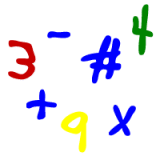Parents and Teachers: Support Ducksters by following us onor.

Kids Math

Ratios, Fractions, and PercentagesAs we discussed in ratios for kids, ratios can be written as fractions. We can do this to help reduce ratios to their simplest form.

Here's an example of using fractions to help reduce the ratio:

Reduce the ratio 6:72 to its simplest form

6:72 can be written as the fraction 6/72
6/72 can be reduced to 3/36 by dividing both the numerator and denominator by 2
3/36 can be further reduced to 1/12 by dividing both the numerator and denominator by 3
1:12 is the simplest form of the ratio

Proportions

We haven't used this term yet, but a proportion is when ratios are equal to each other. Similar to when we have reduced ratios to their simplest form using fractions, we have created ratios that are proportional.

The above example shows a proportion where:

6/72 = 1/12

In this case 6 is to 72 as 1 is to 12. These ratios are proportional and say the same thing.

Percentages

Proportions are often written as percentages.

Here is a simple example:

The following ratios are all proportional:

5:50 6:60 10:100

They all can be reduced to another proportion 1:10. This can be written as a percentage of 10 %. All of the above ratios can be written as 10 %.

Note: in order for a percentage to make sense, the second number or term in the ratio needs to be a total number or the total set number. This is a bit confusing, so we'll describe this concept more in the next section.

Are ratios the same as fractions?

We often write ratios as fractions, especially to help us to do the math, but are they the same as fractions? Generally ratios are best written as fractions when the second term, called the consequent term, is the total of the set.

For example, if we have 8 apples and 12 oranges, our ratio of apples to fruit is 8:20. Written as a fraction this would be 8/20 or 2/5. This means that two fifths of our fruit is apples. This makes sense.

Note: this ratio can also be written as a percentage; 40% of the fruit is apples.

Next let's compare the ratio of apples to oranges which is 8:12. This can be written as the fraction 8/12 and reduced to 2/3. But this fraction doesn't tell us much or make a lot of sense beyond the ratio of apples to oranges. We have 2/3 of what? Doesn't really mean a lot.

You can't really write this as a percentage either. It would be rounded to 67%, but 67% of what? You need the consequent, or second term, to be the total or the number of fruit.

More Algebra Subjects
Algebra glossary
Exponents
Linear Equations - Introduction
Linear Equations - Slope Forms
Order of Operations
Ratios
Ratios, Fractions, and Percentages
Solving Algebra Equations with Addition and Subtraction
Solving Algebra Equations with Multiplication and Division

Back to Kids Math

Back to Kids StudyFollow us onor